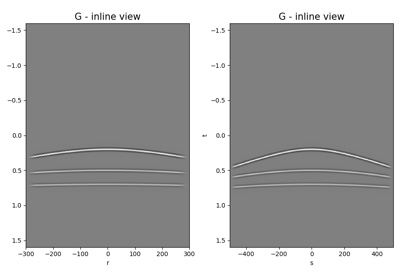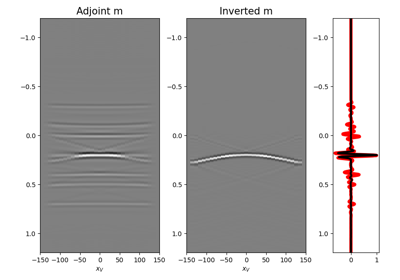# pylops.waveeqprocessing.MDC#

pylops.waveeqprocessing.MDC(G, nt, nv, dt=1.0, dr=1.0, twosided=True, fftengine='numpy', saveGt=True, conj=False, usematmul=False, prescaled=False, name='M')[source]#

Multi-dimensional convolution.

Apply multi-dimensional convolution between two datasets. Model and data should be provided after flattening 2- or 3-dimensional arrays of size $$[n_t \times n_r \;(\times n_{vs})]$$ and $$[n_t \times n_s \;(\times n_{vs})]$$ (or $$2n_t-1$$ for twosided=True), respectively.

Parameters
Gnumpy.ndarray

Multi-dimensional convolution kernel in frequency domain of size $$[n_{f_\text{max}} \times n_s \times n_r]$$

ntint

Number of samples along time axis for model and data (note that this must be equal to $$2n_t-1$$ when working with twosided=True.

nvint

Number of samples along virtual source axis

dtfloat, optional

Sampling of time integration axis $$\Delta t$$

drfloat, optional

Sampling of receiver integration axis $$\Delta r$$

twosidedbool, optional

MDC operator has both negative and positive time (True) or only positive (False)

fftenginestr, optional

Engine used for fft computation (numpy, scipy or fftw)

saveGtbool, optional

Save G and G.H to speed up the computation of adjoint of pylops.signalprocessing.Fredholm1 (True) or create G.H on-the-fly (False) Note that saveGt=True will be faster but double the amount of required memory

conjstr, optional

Perform Fredholm integral computation with complex conjugate of G

usematmulbool, optional

Use numpy.matmul (True) or for-loop with numpy.dot (False) in pylops.signalprocessing.Fredholm1 operator. Refer to Fredholm1 documentation for details.

prescaledbool, optional

Apply scaling to kernel (False) or not (False) when performing spatial and temporal summations. In case prescaled=True, the kernel is assumed to have been pre-scaled when passed to the MDC routine.

namestr, optional

New in version 2.0.0.

Name of operator (to be used by pylops.utils.describe.describe)

Raises
ValueError

If nt is even and twosided=True

MDD

Multi-dimensional deconvolution

Notes

The so-called multi-dimensional convolution (MDC) is a chained operator . It is composed of a forward Fourier transform, a multi-dimensional integration, and an inverse Fourier transform:

$y(t, s, v) = \mathscr{F}^{-1} \Big( \int_S G(f, s, r) \mathscr{F}(x(t, r, v))\,\mathrm{d}r \Big)$

which is discretized as follows:

$y(t, s, v) = \sqrt{n_t} \Delta t \Delta r\mathscr{F}^{-1} \Big( \sum_{i_r=0}^{n_r} G(f, s, i_r) \mathscr{F}(x(t, i_r, v)) \Big)$

where $$\sqrt{n_t} \Delta t \Delta r$$ is not applied if prescaled=True.

This operation can be discretized and performed by means of a linear operator

$\mathbf{D}= \mathbf{F}^H \mathbf{G} \mathbf{F}$

where $$\mathbf{F}$$ is the Fourier transform applied along the time axis and $$\mathbf{G}$$ is the multi-dimensional convolution kernel.

1

Wapenaar, K., van der Neut, J., Ruigrok, E., Draganov, D., Hunziker, J., Slob, E., Thorbecke, J., and Snieder, R., “Seismic interferometry by crosscorrelation and by multi-dimensional deconvolution: a systematic comparison”, Geophysical Journal International, vol. 185, pp. 1335-1364. 2011.

## Examples using pylops.waveeqprocessing.MDC#Multi-Dimensional Convolution

Multi-Dimensional Convolution09. Multi-Dimensional Deconvolution

09. Multi-Dimensional Deconvolution